## 4. 1. 1 Algebra of Linearized Equations

One equation, comprising a matrix line and one right-hand-side vector entry is considered a mathematical entity. It can be viewed as an equation which depends on a number of unknown variables on which the equation linearly depends.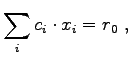(4.1)

where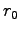denotes the right hand side entry,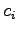denotes the coefficients with which the solution variables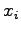are weighted. Alternatively, the entity can be seen as residuum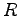that implicitly has to be zero.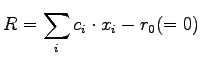(4.2)

This view is perhaps more illustrative, because addition, multiplication, function application, and so forth of residual expression seems to be more natural than the respective application of operations on equations. If the operations are applied to residual expressions, it is always assumed that the expression is followed by a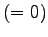. For the sake of simplicity, the matrix line can be seen as line vector and can be written in a matrix formalism: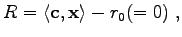(4.3)

where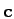denotes the vector of coefficientsand and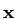denotes the solution variables. Furthermore, the operations used have to provide an algebraic structure. The first and most important requirement on such a structure is closedness. This means, that the result of applying operations on one or more expressions still remains an expression of the same type. Note, if linear (residual) expressions depending on one or more variables are applied to a function the result is still a linear expression.

It can be seen easily that the application of arbitrary functions on linear expressions does not necessarily lead to linearized expressions. Only very few operations (i.e. linear operations) preserve the algebraic structure of a linear equation. This especially holds true for addition and scalar multiplication by which an affine linear algebra is created. Of course, in general, especially when solving non-linear equations such an algebraic structure cannot be preserved.

This problem can be easily fixed, if after each nonlinear operation a subsequent linearization step is performed. It can be shown easily that the number of intermediate linearization steps is not relevant as long as removable discontinuities are avoided.

In the following sections the basic operations are shown. As an example, the method is demonstrated on a simple nonlinear discretized differential equation system. Afterwards the assembly of the system matrix is shown. In a second example, the same calculations are carried out for an eigenvalue equation system.

Michael 2008-01-16